Next: 2.3.3.4 Non-linear Capacitor Up: 2.3.3 Devices Previous: 2.3.3.2 Resistor

### 2.3.3.3 Linear Capacitor

The constitutive relation for a linear capacitor is I = C . dV/dt. For the backward Euler discretization scheme the equations are as follows
 I = G .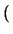V - Vo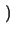(2.35) G =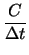(2.36)

The stamp is given as
 yx, y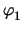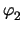f n1 G - G - I n2 - G G I

For thermal capacitors C is substituted by Cth and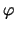by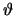, respectively.

Tibor Grasser
1999-05-31